# Normal operator

(diff) ← Older revision | Latest revision (diff) | Newer revision → (diff)

A closed linear operatordefined on a linear subspacethat is dense in a Hilbert spacesuch that, where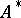is the operator adjoint to. Ifis normal, thenand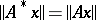for every. Conversely, these conditions guarantee thatis normal. Ifis normal, then so are;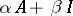for any;when it exists; and if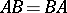, whereis a bounded linear operator, then also.

A normal operator has:

1) the multiplicative decomposition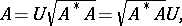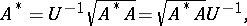whereis a unitary operator which is uniquely determined on the orthogonal complement of the null space ofand;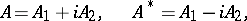where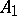andare uniquely determined self-adjoint commuting operators.

The additive decomposition implies that for an ordered pair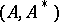there exists a unique two-dimensional spectral function, where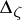is a two-dimensional interval,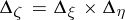,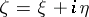, such that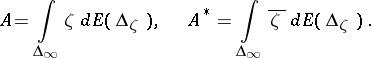The same decomposition also implies that a normal operatoris a function of a certain self-adjoint operator,. Conversely, every function of some self-adjoint operator is normal.

An important property of a normal operatoris the fact that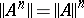, which implies that the spectral radius of a normal operatoris its norm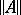. Eigen elements of a normal operator corresponding to distinct eigen values are orthogonal.

How to Cite This Entry:
Normal operator. Encyclopedia of Mathematics. URL: http://encyclopediaofmath.org/index.php?title=Normal_operator&oldid=17283
This article was adapted from an original article by V.I. Sobolev (originator), which appeared in Encyclopedia of Mathematics - ISBN 1402006098. See original article